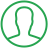# Fibonacci Series Program In Java Using Recursion

Last Updated : Jul 1, 2023In this article we will show you the solution of fibonacci series program in java using recursion, that each digit in the Fibonacci sequence from 0 to 1, 2, 3, 4, 6, 12, 21, 34, and so forth—is equal to the sum of its two predecessors. The fibonacci series' two preceding digits are 0 and 1.

There are two approaches to implement the Fibonacci series in Java: without recursion and utilising the Fibonacci Number.

PreviousNumber and nextNumber in the Fibonacci Recursive Series has initial values of 0 and 1, correspondingly. The Fibonacci While Loop iterates through Maximum Number.

PreviousNumber and nextNumber now have updated values.

The justification is still the same as previously.

Instead of the number being hardcoded inside the Java Fibonacci Series, a user gets prompted to enter it.

The Java function fibonacciRecursion() accepts a number as an argument. because the Java Fibonacci sequence begins with 0, 1, 1, it checks on 0,1,2 and returns 0,1,1.

This method will call itself again if the argument n is greater than 3. The call is placed twice.

Let's look at an example of the Fibonacci Sequence in Java utilising recursion with a 4 as the input.

Java programming is used to calculate the Fibonacci series using both iterative and recursive methods.

Two functions are used in this instance: fibonacci (int number) and fibonacci2 (int number). The first one employs recursion to print the Fibonacci series, whereas the second one makes use of a loop or iteration.

## Step By Step Guide On Fibonacci Series Program In Java Using Recursion :-

```public class TalkersCode{
static int n1 = 0,n2 = 1,n3 = 0;
static void fibbonacci(int count) {
if (count > 0) {
n3 = n1 + n2;
n1 = n2;
n2 = n3;
System.out.print(" " + n3);
fibbonacci(count - 1);
}
}
public static void main(String args[]) {
int count = 5;
System.out.print(n1 + " " + n2);
fibbonacci(count - 2);
}
}```
1. First, we make a public class called TalkersCode.
2. Then we create a static function.
3. After that we count an integer number with n1, and n2, n3.
4. After that we create the Fibonacci count of "+count+" numbers using system.out.println.
5. After that we define the public static void main as a int count.
6. After that we define fibbonacci count numbers using system.out.println.

## Conclusion :-

The Fibonacci series is indeed a set containing natural no where each successive number is the product of the two preceding numbers, as in fn = fn-1 + fn-2.

The first 2 numbers in the Fibonacci numbers always are 1 and 2.

We develop a function to calculate Fibonacci numbers in this Java programme example for the Fibonacci sequence, and then we print those numbers.

I hope this article on fibonacci series program in java using recursion helps you and the steps and method mentioned above are easy to follow and implement.

##About Pragati

Experienced coding content writer who enjoys breaking down complex concepts in programming languages like Java, Python, C, and C++. Over three years of experience producing interesting and relevant content for a variety of entities. Committed to providing concise and easy-to-understand articles that assist readers in easily understanding technology and industry trends in the world of coding and software development.